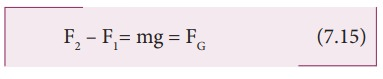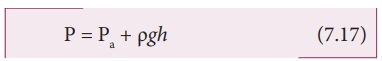Home | | Physics 11th std | Pressure due to fluid column at rest

# Pressure due to fluid column at rest

A mountaineer climbing the mountain is able to experience a decrease in pressure with altitude.

Pressure due to fluid column at rest

A mountaineer climbing the mountain is able to experience a decrease in pressure with altitude. A person jumping into the swimming pool always realizes an increase in pressure with depth below the water surface. In both the cases, the pressure encountered by the mountaineer and diver is usually due to the hydrostatic pressure, because they are due to fluids that are static. In order to understand the increase in pressure with depth below the water surface, consider a water sample of cross sectional area in the form of a cylinder. Let h1 and h2 be the depths from the air-water interface to level 1 and level 2 of the cylinder, respectively as shown in Figure 7.10(a). Let F1 be the force acting downwards on level 1 and F2 be the force acting upwards on level 2, such that, F1 = P1 A and F2 = P2 A Let us assume the mass of the sample to be m and under equilibrium condition, the total upward force (F2) is balanced by the total downward force (F1 + mg), in other words, the gravitational force will act downward which is being exactly balanced by the difference between the force F2 ŌĆō F1.

F2 ŌĆō F1= mg = FG          (7.15)where m is the mass of the water available in the sample element. Let Žü be the density of the water then, the mass of water available in the sample element isHence, gravitational force,

FG = Žü A (h2 ŌĆō h1) g

On substituting the value of W in equation (7.15)

F2=F1+m g ŌćÆ P2A = P1A+ ŽüA(h2-h1)g

Cancelling out A on both sides,

P2 = P1 + Žü(h2 ŌĆō h1)g             (7.16)If we choose the level 1 at the surface of the liquid (i.e., air-water interface) and the level 2 at a depth ŌĆśhŌĆÖ below the surface (as shown in Figure 7.10(b)), then the value of h1 becomes zero (h1 = 0) and in turn P1 assumes the value of atmospheric pressure (say Pa). In addition, the pressure (P2) at a depth becomes P. Substituting these values in equation (7.16), we get

P = Pa + Žügh (7.17)which means, the pressure at a depth h is greater than the pressure on the surface of the liquid, where Pa is the atmospheric pressure which is equal to 1.013 x 105 Pa.

If the atmospheric pressure is neglected or ignored then

P = Žügh       (7.18)For a given liquid, Žü is fixed and g is also constant, then the pressure due to the fluid column is directly proportional to vertical distance or height of the fluid column. This implies, the height of the fluid column is more important to decide the pressure and not the cross sectional or base area or even the shape of the container.

When we talk about liquid at rest, the liquid pressure is the same at all points at the same horizontal level (or same depth). This statement can be demonstrated by an experiment called ŌĆśhydrostatic paradoxŌĆÖ. Let us consider three vessels of different shapes A, B, and C as shown in Figure 7.11. These vessels are connected at the bottom by a horizontal pipe. When they are filled with a liquid (say water), it occupies the same level even though the vessels hold different amounts of water. It is true because the liquid at the bottom of each section of the vessel experiences the same pressure.Study Material, Lecturing Notes, Assignment, Reference, Wiki description explanation, brief detail
11th Physics : UNIT 7 : Properties of Matter : Pressure due to fluid column at rest |